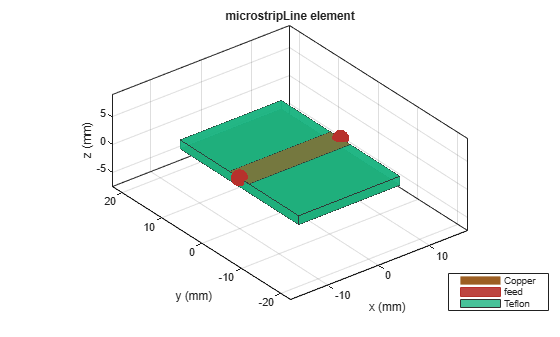# metal

Conductor material

## Syntax

``m = metal(material)``
``m = metal(Name=Value)``

## Description

example

````m = metal(material)` returns the metal used as a conductor in the PCB components. You can specify a material from the `MetalCatalog` (Antenna Toolbox). The default value for material is perfect electric conductor (PEC).```
````m = metal(Name=Value)` returns the metal based on the properties specified by one or more .name-value pairs.```

## Examples

collapse all

Create a microstrip transmission line with copper conductor.

`mline = microstripLine;`

Create a copper metal conductor.

`m = metal('copper')`
```m = metal with properties: Name: 'Copper' Conductivity: 59600000 Thickness: 3.5560e-05 For more materials see catalog ```

Change the microstrip transmission line conductor to copper.

`mline.Conductor = m`
```mline = microstripLine with properties: Length: 0.0200 Width: 0.0050 Height: 0.0016 GroundPlaneWidth: 0.0300 Substrate: [1x1 dielectric] Conductor: [1x1 metal] ```

View the microstrip transmission line.

`show(mline)`## Input Arguments

collapse all

Material from the dielectric catalog, specified as a metal from the `MetalCatalog`. The default material is perfect electric conductor (PEC), which has infinite conductivity and zero thickness.

Example: `'Iron'`

Data Types: `char`

### Name-Value Arguments

Example: `Name='Iron'`

Name of the metal material you want to use as a conductor, specified as a character vector.

Example: `Name='Iron'`

Data Types: `char`

Conductivity of the metal material, specified as a scalar in Siemens per meter(S/m). If you set `'Conductivity'` to `'Inf'`, you must set `'Thickness'` to `'0'`.

Example: `Conductivity=4.8e06`

Data Types: `double`

Thickness of the metal material along the default z-axis, specified as a scalar in meters.

Example: `Thickness=0.26e-6`

Data Types: `double`

## Output Arguments

collapse all

Conductor metal, returned as a metal object.

## Version History

Introduced in R2021a